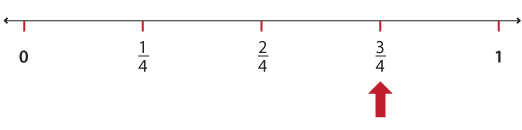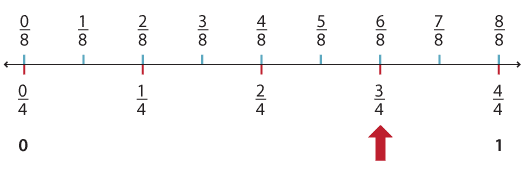### Locating fractions on the number line

The word fraction comes from the Latin frango which means 'I break'. On the number line we 'break' the number line into equal parts.

If we want to locate $$\dfrac{3}{4}$$ on the number line, we start with a number line from 0 to 1. We break the segment between 0 and 1 into 4 equal parts. Each part is one-quarter. We mark $$\dfrac{3}{4}$$ with an arrow.Detailed description

We can also break the segment between 0 and 1 into 8 equal pieces. Each piece is one-eighth. We see that $$\dfrac{6}{8}$$ marks the same place as $$\dfrac{3}{4}$$, so we say that they are equivalent.Detailed description

We can also see that $$\dfrac{1}{4}$$ is equivalent to $$\dfrac{2}{8}$$, $$\dfrac{2}{4}$$ is equivalent to $$\dfrac{4}{8}$$ and $$\dfrac{8}{8}$$ and $$\dfrac{4}{4}$$ are equivalent to 1.

Definition: Two fractions are equivalent if they mark the same point on the number line.